Home > mini projects > Truly random and Pseudorandom Data Generation with Thermal Noise

Truly random and Pseudorandom Data Generation with Thermal Noise

Abstract-In this project, an approach for generation of random data with hardware circuitry from thermal noise is studied. Two hardware circuits are simulated for random data generation. While the first one is designed for truly random data, the later is designed for pseudorandom data generation. A new approach for generating random data with desired probabilities of logic 0 and logic 1 is presented in coherence with the bernoulli distribution. The randomness of generated output data is studied and the applicability of thermal noise is verified. A study of possible applications for these circuits is also carried out. The potential applications of both the methods are studied. Finally, the circuits are simulated to obtain data which are random in nature.

Index Terms-random data, thermal noise, pseudo-random, truly random, bernoulli distribution.

I. INTRODUCTION

In this project, an approach is presented for generation of random data. Truly random data generation is very essential in certain applications. In communication, addition of random noise through channel with the transmitted signal acts as an unnecessary disturbance. This needs to be taken into account during simulations for modelling a practical real life scenario. On the contrary, randomization is purposefully introduced during encryption of data to avoid being intercepted and interpreted. Random number generators have applications in gambling, statistical sampling, computer simulation, cryptog-raphy, completely randomized design, and other areas where producing an unpredictable result is desirable .

True random number generation is the most essential factor in determining the quality of a generated random data. The data in any form shouldn't be deterministic in nature. Broadly we can categorise the process of generation of random data in 3 types- software based, hardware based and mathematical for-mulation . Remarkable advancements have been observed in recent times in the form of algorithms to generate random patterns on computers. In , a new pseudo-random generation method is proposed in a database system. Despite high level of randomness, these algorithms need to start with an initial value, also called as the seed value. Random data generated from hardware on the other hand has potential to be a truly random quantity such as thermal noise as the starting point. An image-based approach to generate truly random numbers from the surface of water bodies such as oceanic bays is presented in . Such techniques can be considered truly random in the sense that no formula or algorithm can predict the future values. Mathematical formulation of random data has been studied traditionally for many years now, but the seed value is still a problem despite the freedom formulations similar to gaussian distribution. provides an account of consistently developing mathematical and statistical methods for random number generation.

This project primarily focuses on generating noise through hardware which can be practically implemented for mimicking the effect of channel noise by introduction of bit errors. When it comes to hardware implementation, noise as a starting point is very popular. Many physical phenomena in nature are observed which are completely random in nature. An innova-tive way of Ferroelectric switching in Hafnium oxide-based ferroelectric field-effect transistors (FeFETs) is studied in  for generating random number. This current project focuses on the thermal noise created at all non-zero temperatures due to the vibration of atoms at the molecular level. Accordingly, 2 circuits are simulated considering practical components for truly random and pseudorandom data generation in respective circuits.

Firstly, a truly random data generator circuit is developed for modelling the noise in a channel during testing of a transceiver system. Channel tends to attenuate and distort a signal and has a tendency to introduce error in the transmitted signal. As the communication distance increases, there is a higher probability for occurrence of error. To model this issue, a provision is made in the circuit to vary the probabilities of occurrence of logic 0 and logic 1 in coherence with the bernoulli distribution. This is a novel take on how the user can control the behaviour of random data according to his need and application. As an example, if the channel needs to be modelled for communication between two places within a city, the amount of error introduced will be less when compared to the error introduction in communication between two faraway cities. This idea is presented with the help of bernoulli distribution that in an event with only 2 possibilities, if a particular logic has a probability p, the other logic will have probability 1-p. This ease in controlling the data is provided with a simple voltage input which can be adjusted by the user according to his preference. Secondly, a circuit is modelled with shift registers to generate random data which becomes periodic after a certain time interval. This particular case has an application in encryption of data, wherein a single plaintext P can be encrypted into many different cipher texts C1, C2, ... Cn and when we encrypt, one of these cipher texts will be selected randomly . Similar scheme can then be employed in the decryption mechanism to map the received data to one of the cipher texts. The specific type of data for the desired application are important. This application and the circuit in consideration are examples of pseudorandom data

generation, since among a set of possible events any event is selected randomly for further processing. In such a set-up, even the receiver has the capability to map the encrypted text to the original text by guessing the particular event used among many available ones.

There exists literature for use of thermal noise for random data generation purpose. To the best of authors' knowledge, this application of bernoulli distribution to control the ran-domly generated data has been presented for the first time. Moreover, most applications for cryptography are software based. In this era of cyber security issues, hardware circuit for introducing randomization is a good step towards tackling the issue of hacking. Rest of the project is presented as follows: Section II presents Architecture of the circuit; Section III presents analysis and simulation results of the designed circuits and finally conclusions from the obtained results are drawn in Section IV. All circuits discussed in this project are designed and simulated using ngspice software.

II. ARCHITECTURE OF THE CIRCUIT

Two unique circuit designs are proposed in this project for random data generation, with their own domain of applica-tions. The truly random data generator focuses on amplifying the thermal noise from the noise generator circuit in the initial stage as in Fig. 1. This amplified noise is comparable to logic 0 and logic 1 levels and is passed through a flip-flop to hold a particular output for a particular clock interval.Fig. 1. Block diagram of truly random data generator circuit.

The second proposed circuit is a design consisting of a basic 4 bit shift register with a feedback. The unique combination of outputs of various stages of this shift register set-up is processed through a digital circuit. This leads to generation of a bit pattern which is random on short intervals but repeats on long duration. This form of bit patterns can be used for encryption and decryption of data on communication channels. The repetitive behaviour enables to recover back the original data transmitted.

A. Truly random data generator

The first circuit (Fig. 2) mainly consist of three stages, viz. practical noise source, amplification stage and quantiser to limit the amplitude in binary voltage level. This circuit generates purely random data for modelling the effects of any communication channel. Moreover, the circuit has a user controlled input to gain a control over the probability of emission of the logic levels. The general structure of the circuit can be represented by Fig. 1. The core concept of this circuit implementation is use of randomly varying voltage across a high value resistor. Thus is called thermal noise in resistor. Thermal noise is a function of three parameters. Its behaviour

Fig. 2. Circuit diagram for truly random data generator.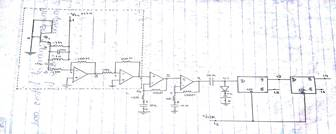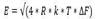Fig. 3. Equation for noise formulation.

is represented by equation in Fig. 3. In the equation, E is RMS Noise strength, R is Resistance value in ohms, K is Boltzmann constant(1.38e-23) V/K), F is Bandwidth of signal and T is temperature in K

The noise generated by resistance is in the order of fV range. Hence a set of cascaded amplifiers with a suitable gain are employed so as to amplify it to a few volts. A special arrangement of a circuit is introduced at this stage to give a DC offset to the amplified noise. This newly generated waveform is now fed as an input to a set of two synchronous D flip-flops. These D flip-flops latch the value of noise when there is a negative transition on their clock input. Hence the noise is sampled randomly. The output of the flip-flop is quantised in two levels i.e. 0 and 5V. The circuit is also provided with a clamper circuit to shift the DC offset of the noise signal after amplification stage. This shifting in DC level has the potential to affect the probabilities of both the logics. This scenario is best depicted by bernoulli distribution, wherein probabilities of neither logic are equal to 0:5. Unequal presence of either logic in a random data can be exploited to represent the intensity of error introduction through the channel.

B. Pseudorandom data generator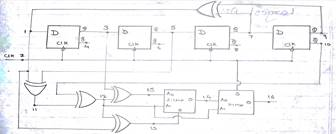Fig. 4. Circuit diagram of pseudorandom data generator.

The circuit designed (Fig. 4) for the random data generator derives its input from a unique combination of its previous outputs. This leads to varying time binary input to the shift register. Further to add more randomness to the output, a digital circuit which goes through variety of states for different input combinations is employed. This will further add random

selection of available data from the circuit. Since the overall circuit is can be represented as a finite state machine it repeats the state after a long interval, hence the name Pseudo Random Data Generator.

III. CIRCUIT ANALYSIS

The designed circuits were simulated to obtain random stream of logic 1 and logic 0. The real challenge lies in the data being truly random. Since the starting point is thermal noise, the results are expected to change randomly every single iteration. Without any disturbance in the initial circuit, the data stream observed is thoroughly random and more importantly, the probabilities of occurrence of logic 0 and logic 1 are almost equal at p = 0:5.

A. Truly random data analysis

The noise from the noise generator circuit is significantly amplified to be comparable to logic level for data transmission. A sample of random noise is presented in Fig. 8. Thereafter, the amplified noise is fed to flip-flop to map the output to either logic 0 or logic 1. The output of the random data is presented in Fig. 7. There is a clamper circuit between the flip-flop and the amplification stage. A user provided voltage is present in the clamper circuit which can move the DC offset level of the noise to vary the probabilities of occurrence of the logics. As mentioned earlier, this change in probabilities can be best represented by bernoulli distribution given as in Fig.

5. A sample graph for a bernoulli distribution with unequal probabilities is presented in Fig. 6.Fig. 5. Equation for bernoulli distribution.Fig. 6. Graph for bernoulli distribution.

The circuit is simulated for a negative applied voltage at the clamper circuit. This shifts down the DC offset level. Accordingly, the probability of logic 0 increases. This is clear from Fig. 9

B. Pseudorandom data analysis

The pseudorandom data generator output is random and periodic to some extent. This implies that the circuit being similar to a finite state machine, the circuit starts with a

Fig. 7. Graph for truly random output data.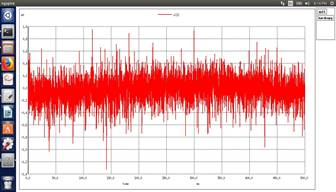Fig. 8. Thermal noise from the noise generator circuit after amplification.

random logic and eventually follows a certain standard path. This can be implemented for encryption and decryption since the finite state logics will be somehow definite if the circuit for random data generation and reception is similar at both the ends. In addition, the receiving circuit will have to try certain combination for decrypting the data, but the number of combinations will be fixed and the data will accordingly be decrypted. Fig. 10 presents the output random data for the pseudorandom data generator circuit. After detailed study and analysis of the behaviour of the pseudo random generator was carried out for wide range of input frequencies. The circuit can perform consistently for a large number of operating fre-quency. The clock inputs to the flip-flops decide the operating frequency of the system. If the entire system is considered as a black box, then care has been taken that the final output of the black box will respond according to the input clock transitions. The repetitive behaviour of the circuit can be controlled as per application be slight modification in the hardware of the system or by cascading systems.

C. Applications

The proposed idea of random data and its generation method finds various applications in the field of communication, data security and in many engineering and statistical applications. The concept of Pseudo random code generation is widely used in common applications like sensor networks. In a sensor network it is essential to check the status of each node or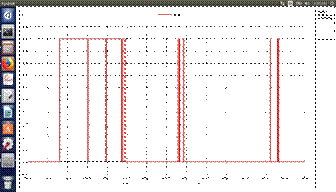Fig. 9. Random data after shifting DC offset level.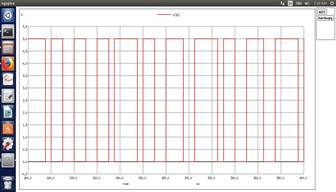Fig. 10. Graph for pseudorandom output data.

the output data bits. The pseudorandom data generator starts with a random logic level and acts as a finite state machine with an arbitrary logic which cannot be precisely determined. On every positive edge of the clock the D flip-flop latches the input noise value and decides the output. The output tends to repeat after a long interval of time. This interval being large, it apparently behaves like random data.IV. CONCLUSION

In this project an approach was proposed for truly random and pseudorandom data generation in 2 respectively designed

circuits. The truly random circuit amplified noise and accord-ingly mapped the same with logic levels with the help of D

flip-flop. Random data generator decides its output based on the noise value it receives. The clamper circuit at the input

of D flipflop gives a DC Offset to the amplified noise. Thus giving the user a full control of adjusting the probabilities of